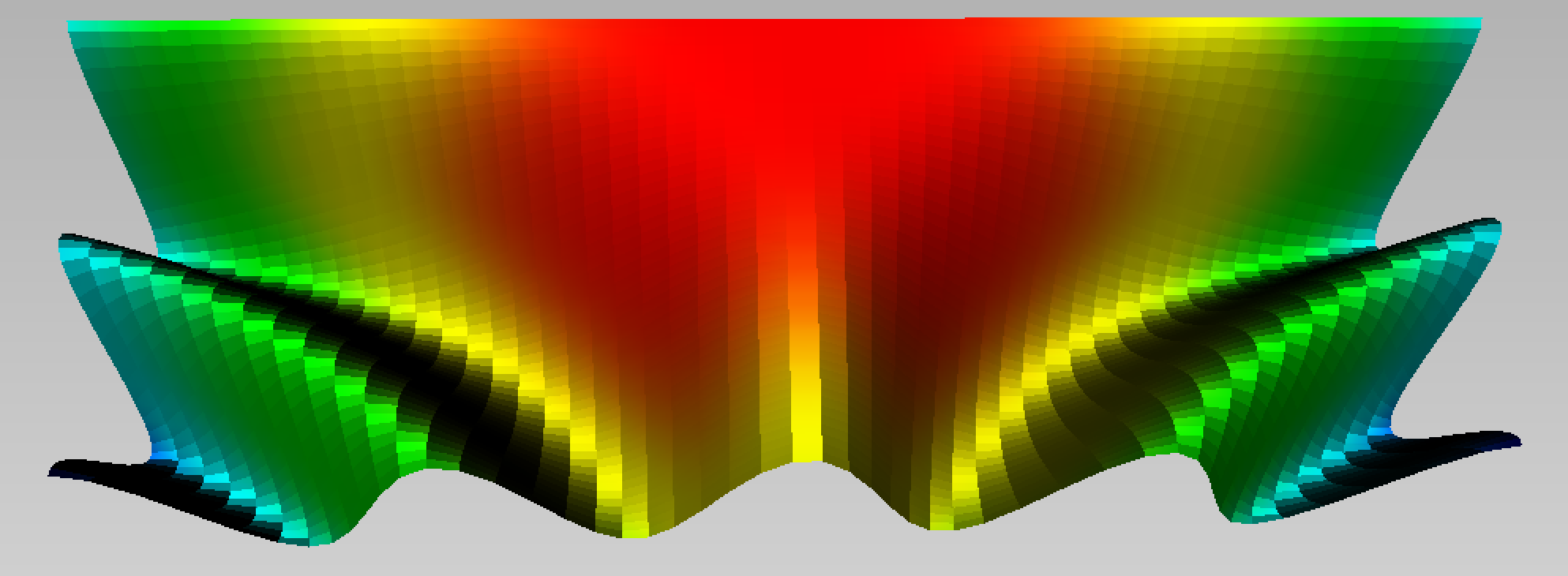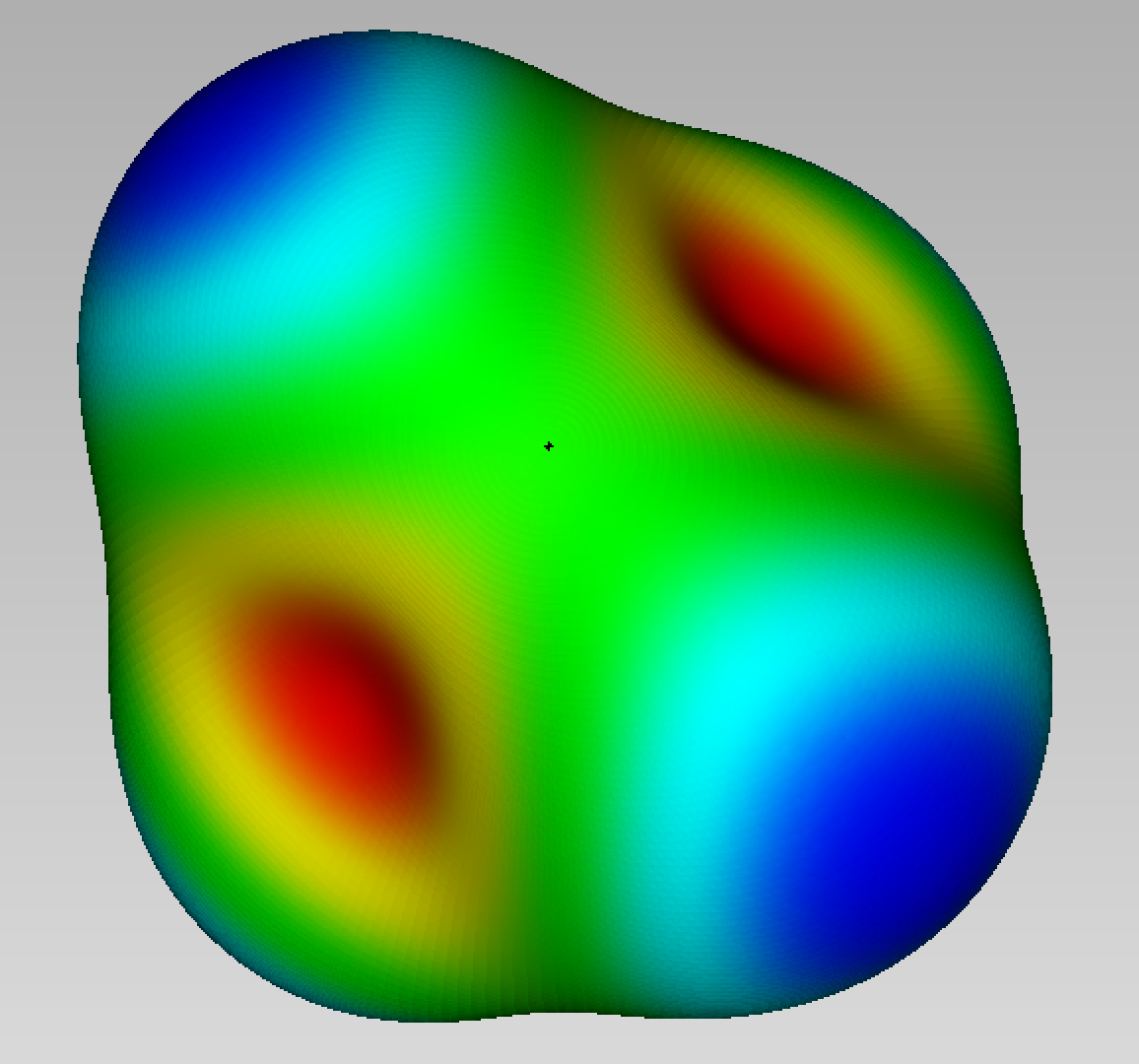# Parametric plots

Axl.parametricFunction

Plot the parametric surface f: (u,v) -> [x,y,z] for u in the interval X, v in the interval Y.

parametric((u,v)->[u,v,cos(2*u*v)], 0.0 => 2.0, -pi => pi, field=DistField(0.0,0.0,0.0))

Plot the parametric surface f: (u,v) -> [x,y,z] for u in the interval X, v in the interval Y.

parametric((u,v)->[u,v,cos(2*u*v)], 0.0 => 2.0, -pi => pi, field=DistField(0.0,0.0,0.0))Axl.polarFunction

Plot the radius function r = f(x,y,z) on the unitary sphere.

polar((x,y,z)->cos(x*y*z+1.0), 200, field=DistField())